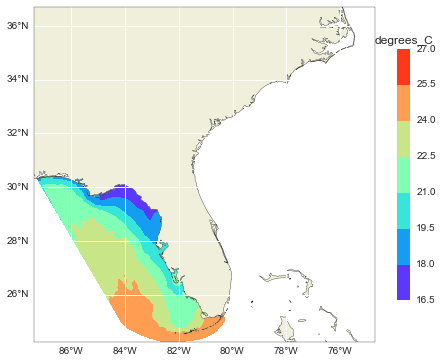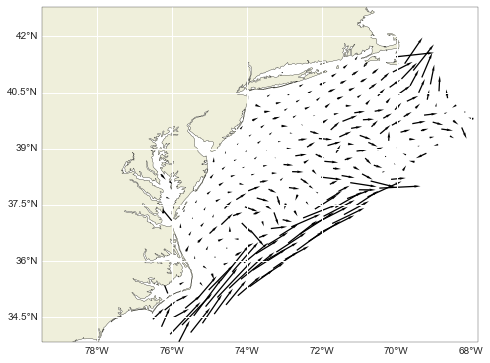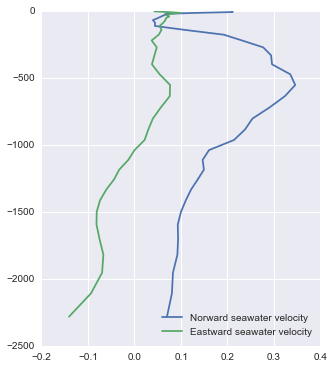How well does iris "speak" Ocean Models?

The problem

Iris works great when loading 1D coordinates cube datasets mapped to a "regular" rectangular grid. But modern ocean models rarely falls into that description, they usually have 2D coordinates mapped to curvilinear staggered grids.

So how well does iris perform with modern numerical ocean models? How about the grids types? Does iris load staggered, unstructured and/or rotated grids? And the dimensionless vertical coordinates? Are they recognized and build automatically?

This post tries to provide a few answers (and some workarounds) to the questions above.

2D coordinates

To my disappointment 2D coordinates are a second class citizen in iris. The dataset can be loaded, but virtually all methods will fail.

In :
import iris
iris.FUTURE.netcdf_promote = True

url = ('http://omgsrv1.meas.ncsu.edu:8080/thredds/dodsC/fmrc/'
'sabgom/SABGOM_Forecast_Model_Run_Collection_best.ncd')

In :
print('X and Y dimensions are: {!r}'.format(cube.coord(axis='X').ndim,
cube.coord(axis='Y').ndim))
X and Y dimensions are: 2

(Note the iris.FUTURE.netcdf_promote = True that promotes netCDF formula terms, like sea surface height, to cubes. This behavior will be default in future versions of iris. For more information on this see PR 1159.)

In :
from iris import Constraint

bbox = [-87.40, 24.25, -74.70, 36.70]
lat = Constraint(latitude=lambda cell: bbox <= cell < bbox)
lon = Constraint(longitude=lambda cell: bbox <= cell <= bbox)

try:
cube = cube.extract(lon & lat)
except Exception as e:
print(e)
Cannot apply constraints to multidimensional coordinates

There is a work around, that I found in the mailing list, to extract a 2D coords subregion with iris:

In :
import numpy as np
from oceans import wrap_lon180

minmax = lambda x: (np.min(x), np.max(x))

def bbox_extract_2Dcoords(cube, bbox):
"""
Extract a sub-set of a cube inside a lon, lat bounding box
bbox=[lon_min lon_max lat_min lat_max].
NOTE: This is a work around too subset an iris cube that has
2D lon, lat coords.

"""
lons = cube.coord('longitude').points
lats = cube.coord('latitude').points
lons = wrap_lon180(lons)

inregion = np.logical_and(np.logical_and(lons > bbox,
lons < bbox),
np.logical_and(lats > bbox,
lats < bbox))
region_inds = np.where(inregion)
imin, imax = minmax(region_inds)
jmin, jmax = minmax(region_inds)
return cube[..., imin:imax+1, jmin:jmax+1]

sub_cube = bbox_extract_2Dcoords(cube, bbox)

print('Original {} and new {} horizontal shapes'.format(cube.shape[1:],
sub_cube.shape[1:]))
Original (320, 440) and new (293, 265) horizontal shapes

Almost all of iris methods, that have something to do with the horizontal coordinates, will fail and a similar workaround will be necessary. Here is another example to use a KDTree to search for nearest points on 2D coords.

Unstructured grids

Full support for unstructured grids will soon be available via pyugrid. See PRs #1359 and #1235.

Bare in mind that pyugrid is not needed to load, subset, search for points and etc. Most of the cube high level stuff already works with unstructured grids. You will need to pay special attention when interpolating and plotting though.

In :
url = 'http://crow.marine.usf.edu:8080/thredds/dodsC/FVCOM-Nowcast-Agg.nc'

cube = cube[-1, 0, ...]  # Latest time step and surface slice.
In :
import numpy.ma as ma
import matplotlib.tri as tri
import matplotlib.pyplot as plt

from scipy.spatial import Delaunay

import cartopy.crs as ccrs
from cartopy.feature import NaturalEarthFeature, COLORS
from cartopy.mpl.gridliner import LONGITUDE_FORMATTER, LATITUDE_FORMATTER

LAND = NaturalEarthFeature('physical', 'land', '10m', edgecolor='face',
facecolor=COLORS['land'])

fig, ax = plt.subplots(figsize=(8, 6),
subplot_kw=dict(projection=ccrs.PlateCarree()))

ax.set_extent([bbox, bbox, bbox, bbox])
ax.coastlines(resolution='10m')
gl = ax.gridlines(draw_labels=True)
gl.xlabels_top = gl.ylabels_right = False
gl.xformatter = LONGITUDE_FORMATTER
gl.yformatter = LATITUDE_FORMATTER

lon = cube.coord(axis='X').points
lat = cube.coord(axis='Y').points
nv = Delaunay(np.c_[lon, lat]).vertices
triang = tri.Triangulation(lon, lat, triangles=nv)
cs = ax.tricontourf(triang, cube.data, cmap=plt.cm.rainbow)
cbar = fig.colorbar(cs, extend='both', shrink=0.75)
ctitle = cbar.ax.set_title(cube.units)These three lines

lon = cube.coord(axis='X').points
lat = cube.coord(axis='Y').points
nv = Delaunay(np.c_[lon, lat]).vertices

re-construct the grid connectivity information that was already in the file/url. Iris does not know about it and therefore does not load nor preserve it automatically during operations. Pyugrid, on the other hand, does know what to do with that information. Hopefully unstructured grids with iris will get a lot easier in the near future.

Staggered grids

Staggered grid models, like ROMS, can be very challenging to load when using high level tools like iris. In fact, it is less awkward doing everything at a low level or "manually" with the raw netCDF4 interface.

In :
url = ('http://tds.marine.rutgers.edu/thredds/dodsC/roms/espresso/2013_da/'
'his_Best/ESPRESSO_Real-Time_v2_History_Best_Available_best.ncd')

angle = iris.load_cube(url, 'angle between XI-axis and EAST')

lon = angle.coord(axis='X')
lat = angle.coord(axis='Y')

# Latest time step at the surface (Yes, ROMS surface is at -1!)
u = u[-1, -1, ...]
v = v[-1, -1, ...]
In :
for var in [u, v, lon, lat, angle]:
print('{} shape {}'.format(var.name(), var.shape))
eastward_sea_water_velocity shape (82, 129)
northward_sea_water_velocity shape (81, 130)
longitude shape (82, 130)
latitude shape (82, 130)
angle between XI-axis and EAST shape (82, 130)

Note that the shapes do not match. To explain that I could send you to the ROMS documentation page, or I could show off my tikz skills ;-)

In :
%%tikz -s 200,200 -sc 1.2 -f svg
\draw [style=help lines, step=2](-0.5, -0.5) grid (+2.5, +2.5);

\draw [black](+0, +2) node[anchor=south east] {$\Psi_{i, j+1}$};
\draw [blue] (+1, +2) node[anchor=south] {$v_{i, j+1}$};
\draw [black](+2, +2) node[anchor=south west] {$\Psi_{i+1, j+1}$};

\draw [red]  (+0, +1) node[anchor=east] {$u_{i, j}$};
\draw [green](+1, +1) node {$\rho_{i, j}$};
\draw [red]  (+2, +1) node[anchor=west] {$u_{i+1, j}$};

\draw [black](+0, +0) node[anchor=north east] {$\Psi_{i, j}$};
\draw [blue] (+1, +0) node[anchor=north] {$v_{i, j}$};
\draw [black](+2, +0) node[anchor=north west] {$\Psi_{i+1, j}$};

The velocities $u$ and $v$ are defined on different grids. We have to interpolate/average them to either the $\rho$ points (where salinity and temperature are defined) or the $\psi$ (in theory the proper grid for vector quantities). Here is a full grid scheme.

In :
%%tikz -s 400,400 -sc 1.2 -f svg
\draw [style=help lines, step=2](-0.5, -0.5) grid (+6.5, +6.5);

\draw [green] (-2, +7) node[anchor=west] {M};
\draw [green] (-1, +7) node {$\rho$};
\draw [green] (+1, +7) node {$\rho$};
\draw [green] (+3, +7) node {$\rho$};
\draw [green] (+5, +7) node {$\rho$};

\draw [red] (+0, +7) node {$u$};
\draw [red] (+2, +7) node {$u$};
\draw [red] (+4, +7) node {$u$};
\draw [red] (+6, +7) node {$u$};

\draw [blue] (-2, +6) node[anchor=east] {M};
\draw [blue] (-1, +6) node {$v$};
\draw [blue] (+1, +6) node {$v$};
\draw [blue] (+3, +6) node {$v$};
\draw [blue] (+5, +6) node {$v$};

\draw [green] (-2, +5) node[anchor=west] {M-1};
\draw [green] (-1, +5) node {$\rho$};
\draw [green] (+1, +5) node {$\rho$};
\draw [green] (+3, +5) node {$\rho$};
\draw [green] (+5, +5) node {$\rho$};

\draw [red] (+0, +5) node {$u$};
\draw [red] (+2, +5) node {$u$};
\draw [red] (+4, +5) node {$u$};
\draw [red] (+6, +5) node {$u$};

\draw [blue] (-2, +4) node[anchor=east] {M-1};
\draw [blue] (-1, +4) node {$v$};
\draw [blue] (+1, +4) node {$v$};
\draw [blue] (+3, +4) node {$v$};
\draw [blue] (+5, +4) node {$v$};

\draw [green] (-2, +3) node[anchor=west] {M-2};
\draw [green] (-1, +3) node {$\rho$};
\draw [green] (+1, +3) node {$\rho$};
\draw [green] (+3, +3) node {$\rho$};
\draw [green] (+5, +3) node {$\rho$};

\draw [red] (+0, +3) node {$u$};
\draw [red] (+2, +3) node {$u$};
\draw [red] (+4, +3) node {$u$};
\draw [red] (+6, +3) node {$u$};

\draw [blue] (-2, +2) node[anchor=east] {M-2};
\draw [blue] (-1, +2) node {$v$};
\draw [blue] (+1, +2) node {$v$};
\draw [blue] (+3, +2) node {$v$};
\draw [blue] (+5, +2) node {$v$};

\draw [green] (-2, +1) node[anchor=west] {M-3};
\draw [green] (-1, +1) node {$\rho$};
\draw [green] (+1, +1) node {$\rho$};
\draw [green] (+3, +1) node {$\rho$};
\draw [green] (+5, +1) node {$\rho$};

\draw [red] (+0, +1) node {$u$};
\draw [red] (+2, +1) node {$u$};
\draw [red] (+4, +1) node {$u$};
\draw [red] (+6, +1) node {$u$};

\draw [blue] (-2, +0) node[anchor=east] {M-3};
\draw [blue] (-1, +0) node {$v$};
\draw [blue] (+1, +0) node {$v$};
\draw [blue] (+3, +0) node {$v$};
\draw [blue] (+5, +0) node {$v$};

\filldraw [color=gray](0, 0) circle (.1);
\filldraw [color=gray](0, 2) circle (.1);
\filldraw [color=gray](0, 4) circle (.1);
\filldraw [color=gray](0, 6) circle (.1);
\filldraw [color=gray](2, 0) circle (.1);
\filldraw [color=gray](2, 2) circle (.1);
\filldraw [color=gray](2, 4) circle (.1);
\filldraw [color=gray](2, 6) circle (.1);
\filldraw [color=gray](4, 0) circle (.1);
\filldraw [color=gray](4, 2) circle (.1);
\filldraw [color=gray](4, 4) circle (.1);
\filldraw [color=gray](4,6) circle (.1);
\filldraw [color=gray](6,0) circle (.1);
\filldraw [color=gray](6,2) circle (.1);
\filldraw [color=gray](6,4) circle (.1);
\filldraw [color=gray](6,6) circle (.1);

I am not sure what is the best approach to get a shape consistent dataset. And there is nothing on CF convention about staggered grids (yet) to help. I will use the shrink, function from octant, to create variables with matching shapes. Why shrink? Because it is written in python ;-)

In :
def shrink(a, b):
"""Return array shrunk to fit a specified shape by trimming or averaging."""
if isinstance(b, np.ndarray):
if not len(a.shape) == len(b.shape):
raise Exception('Input arrays must have the same number of'
'dimensions')
a = shrink(a, b.shape)
b = shrink(b, a.shape)
return (a, b)

if isinstance(b, int):
b = (b,)

if len(a.shape) == 1:  # 1D array is a special case
dim = b[-1]
while a.shape > dim:  # Only shrink a.
if (dim - a.shape) >= 2:  # Trim off edges evenly.
a = a[1:-1]
else:  # Or average adjacent cells.
a = 0.5*(a[1:] + a[:-1])
else:
for dim_idx in range(-(len(a.shape)), 0):
dim = b[dim_idx]
a = a.swapaxes(0, dim_idx)  # Put working dim first
while a.shape > dim:  # Only shrink a
if (a.shape - dim) >= 2:  # trim off edges evenly
a = a[1:-1, :]
if (a.shape - dim) == 1:  # Or average adjacent cells.
a = 0.5*(a[1:, :] + a[:-1, :])
a = a.swapaxes(0, dim_idx)  # Swap working dim back.
return a
In :
# Remove the grid borders.
angle = angle[1:-1, 1:-1]
lon = lon[1:-1, 1:-1]
lat = lat[1:-1, 1:-1]

# Shrink u and v to the grid shape.
u = shrink(u.data, angle.shape)
v = shrink(v.data, angle.shape)
In :
for key, var in dict(u=u, v=v, lon=lon, lat=lat, angle=angle).items():
print('{} shape {}'.format(key, var.shape))
lat shape (80, 128)
angle shape (80, 128)
lon shape (80, 128)
u shape (80, 128)
v shape (80, 128)

All shapes are consistent now! But the process to get here was quite cumbersome, and the velocities ($u$, $v$) are no longer cubes, just plain ndarrays. To use iris high level stuff we would have to reconstruct the cube.

Iris developers are aware of the issue, and some actions have already been taken to allow users to load the data and take advantage of the cube object.

Iris cube.regrid() could save us from all that, but regridding does not work with 2D coordinates :-(

Rotated grids

Like with the staggered grids, iris has no automatic "action" on angles when loading the dataset. Unlike the staggered grids there is a CF convention on how to store the angle. And the convention metadata tells us what is needed to get an un-rotated grid.

<entry id="angle_of_rotation_from_east_to_x">
<canonical_units>degree</canonical_units>
<grib></grib>
<amip></amip>
<description>The quantity with standard name angle_of_rotation_from_east_to_x is the angle, anticlockwise reckoned positive, between due East and (dr/di)jk, where r(i,j,k) is the vector 3D position of the point with coordinate indices (i,j,k).  It could be used for rotating vector fields between model space and latitude-longitude space.</description>
</entry>

Here is a workaround:

In :
def rot2d(x, y, ang):
"""Apply the rotation.
https://github.com/hetland/octant/blob/b1163e5f462bf954088056aca009fd3f7cb51e86/octant/tools.py#L26
"""
xr = x * np.cos(ang) - y * np.sin(ang)
yr = x * np.sin(ang) + y * np.cos(ang)
return xr, yr

u, v = rot2d(u, v, angle.data)
In :
fig, ax = plt.subplots(figsize=(8, 6),
subplot_kw=dict(projection=ccrs.PlateCarree()))

ax.set_extent([lon.points.min(), lon.points.max(), lat.points.min(), lat.points.max()])
ax.coastlines(resolution='10m')
gl = ax.gridlines(draw_labels=True)
gl.xlabels_top = gl.ylabels_right = False
gl.xformatter = LONGITUDE_FORMATTER
gl.yformatter = LATITUDE_FORMATTER

n = 5
q = ax.quiver(lon.points[::n, ::n], lat.points[::n, ::n],
u[::n, ::n], v[::n, ::n], color='black', **kw)Ocean Dimensionless Vertical Coordinates

The last topic on this post: iris Ocean Dimensionless Vertical Coordinates (ODVC) factories. Right now iris implements only Ocean Sigma over Z. Ocean Sigma over Z is rarely used, I have no idea why iris developers chose it to start! For a list of more common ones see here. (Those are already available via #1304)

Note that the current version of iris will raise an Exception when trying to create the derived coordinate with insufficient formula terms. But staggered grids do not have the formula terms defined in all grids. So... Congratulations! Two issues in one!!.

PR #1485 will change changed the Exception into a warning. So iris loads the data failing only to construct the vertical coordinate for variables that do not have the formula terms properly specified.

Take a look at this notebook to see side-by-side Matlab/Python examples for ocean sigma coordinate, ocean s-coordinate, ocean sigma over z coordinate (all CF-1.6). The notebook also includes examples for ocean s coordinate g1 (sg1) and ocean s coordinate g2 (sg2), that will be included in CF-1.7.

Before someone asks why implement sg1 and sg2, that are not in the current CF conventions yet, and why leave ocean double sigma coordinate out, well... I could not find a single dataset with ocean double sigma coordinate, but every modern ROMS output is either sg1 or sg2.

The last workaround example show how to use iris promote, to extract all the extra formula terms, need to construct the ODVC for $u$ and $v$:

In :
url = ('http://tds.marine.rutgers.edu/thredds/dodsC/roms/espresso/2013_da/avg_Best/'
'ESPRESSO_Real-Time_v2_Averages_Best_Available_best.ncd')

print(len(cubes))
38

In :
from iris.fileformats.cf import reference_terms

reference_terms['ocean_s_coordinate_g1'] = ['s', 'c', 'eta', 'depth', 'depth_c']

print(len(cubes))
43

In :
t = cubes.extract('sea_water_potential_temperature')
u = cubes.extract('eastward_sea_water_velocity')
v = cubes.extract('northward_sea_water_velocity')

# Latest time step.
t = t[-1, ...]
u = u[-1, ...]
v = v[-1, ...]

ROMS only specify eta at $\rho$ points. So no derived coordinate for $u$ and $v$.

In :
u.derived_coords, v.derived_coords
Out:
((), ())

But things work nicely for properties at the $\rho$ points, like temperature.

In :
derived_coords = t.derived_coords

print('{} ({})'.format(derived_coords.name(), derived_coords.units))
sea_surface_height_above_reference_ellipsoid (meter)

Now let's build z for $u$ and $v$ manually:

In :
# Formula terms.
s = u.coord('ocean_s_coordinate_g1').points
c = u.coord('S-coordinate stretching curves at RHO-points').points
eta = cubes.extract('sea_surface_height').data.squeeze()
depth = cubes.extract('sea_floor_depth').data
depth_c = cubes.extract('S-coordinate parameter, critical depth').data
# depth_c is shown by print(u), but I cannot access it.
In :
# Remove the grid borders.
angle = angle[1:-1, 1:-1]
lon = lon[1:-1, 1:-1]
lat = lat[1:-1, 1:-1]

# One shape to rule them all and in the plot bind them!
shape = (s.shape, angle.shape, angle.shape)

u = shrink(u.data, shape)
v = shrink(v.data, shape)
depth = shrink(depth, shape)
eta = shrink(eta, shape)
In :
S = (depth_c * s)[..., None, None] + (depth - depth_c) * c[..., None, None]
z = S + eta * (1 + S / depth)

and plot the vertical profile of the velocities:

In :
fig, ax = plt.subplots(figsize=(5, 6))
l1, = ax.plot(v[:, 30, 80], z[:, 30, 80], label='Norward seawater velocity')
l2, = ax.plot(u[:, 30, 80], z[:, 30, 80], label='Eastward seawater velocity')
ax.grid(True)
leg = ax.legend(loc='lower right')If iris devs find an easier way to get a automagically shape-consistent cube from staggered grids we will be able to say MatlabTM+snctools goodbye forever!

Iris is evolves fast. It is worth mentioning that many of issues I raised here are under iris devs radar for the next version: Iris-2.

I know that there are several projects out there that, like iris, are great tools for reading and processing ocean netCDF data. Some are ocean model specific, like octant, others are full CDMs like paegan. However, I would like too see more projects like gridfill, eofs and windspharm. These 3 projects take either a ndarray or an iris cube object as its input. Taking advantage of the cube rich metadata and methods, whilst avoiding re-inventing the wheel.

In :
from IPython.core.display import HTML

HTML(html)
Out:

This post was written as an IPython notebook. It is available for download or as a static html.